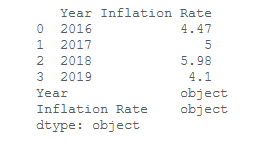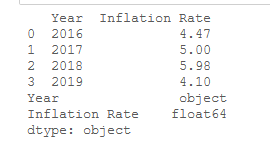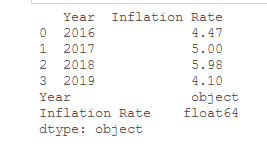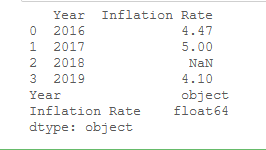# How to Convert Strings to Floats in Pandas DataFrame?

In this article, we’ll look at different ways in which we can convert a string to a float in a pandas dataframe. Now, let’s create a Dataframe with ‘Year’ and ‘Inflation Rate’ as a column.

## Python3

 `# importing pandas library ` `import` `pandas as pd ` ` `  `# dictionary ` `Data ``=` `{``'Year'``: [``'2016'``, ``'2017'``,  ` `                 ``'2018'``, ``'2019'``], ` `        ``'Inflation Rate'``: [``'4.47'``, ``'5'``,  ` `                           ``'5.98'``, ``'4.1'``]} ` `# create a dataframe ` `df ``=` `pd.DataFrame(Data) ` ` `  `# show the dataframe ` `print` `(df) ` ` `  `# show the datatypes ` `print``(df.dtypes)`

Output:Method 1: Using DataFrame.astype().

The method is used to cast a pandas object to a specified dtype.

Syntax: DataFrame.astype(self: ~ FrameOrSeries, dtype, copy: bool = True, errors: str = ‘raise’)

Returns: casted: type of caller

Example: In this example, we’ll convert each value of ‘Inflation Rate’ column to float.

## Python3

 `# importing pandas library ` `import` `pandas as pd ` ` `  `# dictionary ` `Data ``=` `{``'Year'``: [``'2016'``, ``'2017'``,  ` `                 ``'2018'``, ``'2019'``], ` `        ``'Inflation Rate'``: [``'4.47'``, ``'5'``,  ` `                           ``'5.98'``, ``'4.1'``]} ` `# create a dataframe ` `df ``=` `pd.DataFrame(Data) ` ` `  `# converting each value  ` `# of column to a string ` `df[``'Inflation Rate'``] ``=` `df[``'Inflation Rate'``].astype(``float``) ` ` `  `# show the dataframe ` `print``(df) ` ` `  `# show the datatypes ` `print` `(df.dtypes) `

Output:Method 2: Using pandas.to_numeric() function.

The function is used to convert the argument to a numeric type.

Syntax: pandas.to_numeric(arg, errors=’raise’, downcast=None)

Returns: numeric if parsing succeeded. Note that the return type depends on the input. Series if Series, otherwise ndarray.

Example 1: In this example, we’ll convert each value of ‘Inflation Rate’ column to float.

Code:

## Python3

 `# importing pandas library ` `import` `pandas as pd ` ` `  `# creating a dictionary ` `Data ``=` `{``'Year'``: [``'2016'``, ``'2017'``,  ` `                 ``'2018'``, ``'2019'``], ` `          ``'Inflation Rate'``: [``'4.47'``, ``'5'``,  ` `                             ``'5.98'``, ``'4.1'``]} ` `# create a dataframe ` `df ``=` `pd.DataFrame(Data) ` ` `  `# converting each value of column to a string ` `df[``'Inflation Rate'``] ``=` `pd.to_numeric(df[``'Inflation Rate'``]) ` ` `  `# show the dataframe ` `print``(df) ` ` `  `# show the data types ` `print` `(df.dtypes) `

Output:Example 2: Sometimes, we may not have a float value represented as a string. So, pd.to_numeric() function will show an error. To remove this error, we can use errors=’coerce’, to convert the value at this position to be converted to NaN

Code

## Python3

 `# importing pandas as pd ` `import` `pandas as pd ` ` `  `# dictionary ` `Data ``=` `{``'Year'``: [``'2016'``, ``'2017'``, ` `                 ``'2018'``, ``'2019'``], ` `         ``'Inflation Rate'``: [``'4.47'``, ``'5'``,  ` `                           ``'No data'``, ``'4.1'``]} ` ` `  `# create a dataframe ` `df ``=` `pd.DataFrame(Data) ` ` `  `# converting each value of column to a string ` `df[``'Inflation Rate'``] ``=` `pd.to_numeric(df[``'Inflation Rate'``], ` `                                     ``errors ``=` `'coerce'``) ` ` `  `# show the dataframe ` `print``(df) ` ` `  `# show the data types ` `print` `(df.dtypes) `

Output:Note: String data type shows as an object.

Attention geek! Strengthen your foundations with the Python Programming Foundation Course and learn the basics.

To begin with, your interview preparations Enhance your Data Structures concepts with the Python DS Course.

My Personal Notes arrow_drop_upCheck out this Author's contributed articles.

If you like GeeksforGeeks and would like to contribute, you can also write an article using contribute.geeksforgeeks.org or mail your article to contribute@geeksforgeeks.org. See your article appearing on the GeeksforGeeks main page and help other Geeks.

Please Improve this article if you find anything incorrect by clicking on the "Improve Article" button below.

Article Tags :

Be the First to upvote.

Please write to us at contribute@geeksforgeeks.org to report any issue with the above content.